Back to List

# Evaluation curves for object detection algorithms in medical imagesNov 3, 2021 — 15 min read

Thijs Kooi

Safety critical applications of artificial intelligence, like computer aided detection; the detection of abnormalities in medical images, require careful evaluation. In some situations, for example when a system would operate autonomously, we may be satisfied if it simply classifies the case correctly. If there is a human in the loop however, it would be better if the system also marks the correct location of the abnormality in the image. Even if the eventual label is correct, an artificial intelligence system that marks a lesion in the left lung, when it is actually in the right does not look very intelligent and may only disturb the reader, not improve them. Additionally, for diagnosing and improving the model we need to know exactly what is going wrong, not only if the performance is poor.

There is a long history of research on ways to evaluate detection systems for medical images [1, 2, 3, 4, 5, 6] , but usage in practice varies. Some of these measures were developed with a human reader that marks lesions in mind. Evaluating a deep neural net can be quite different. For example, a typical object detection pipeline consists of multiple stages (shown in figure 1), where initial proposals are filtered using techniques like non-maximum suppression. If any of these parameters are poorly calibrated, this can easily result in dozens of proposals for a case. Humans, on the other hand, are not likely to mark 50 regions in a mammogram as suspicious.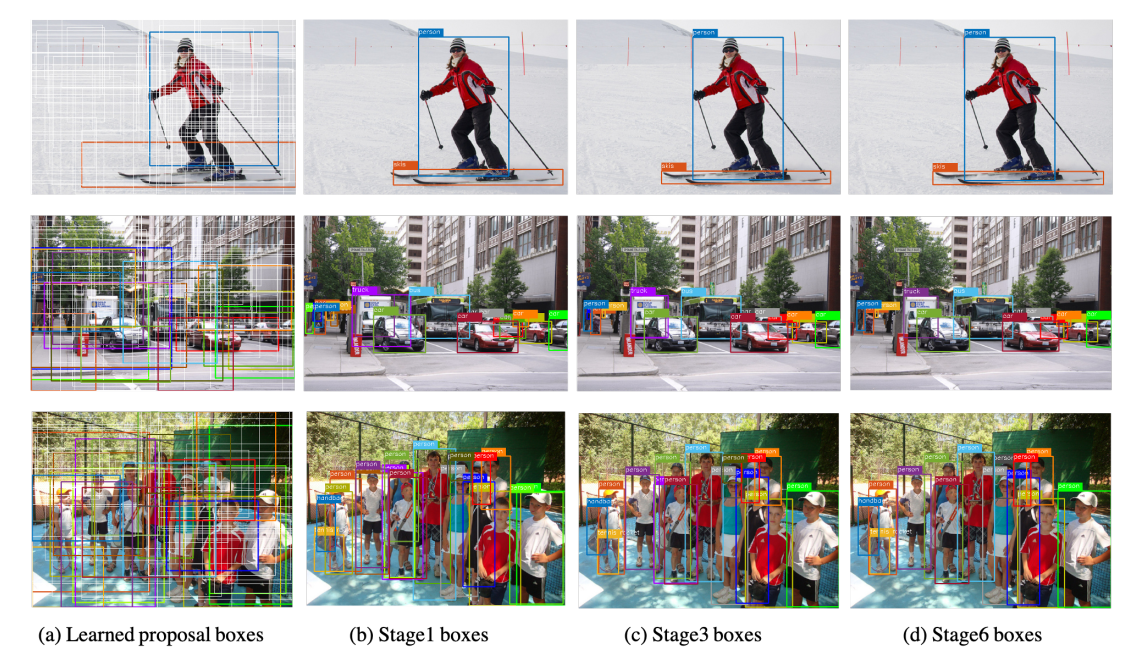This post aims to give a simple summary of the key concepts of several curves specifically used for the evaluation of computer aided detection systems (object detection in medical images). We assume the reader is familiar with basic concepts of receiver operating characteristic (ROC) curves and its properties, if not, please refer to  for an excellent introduction.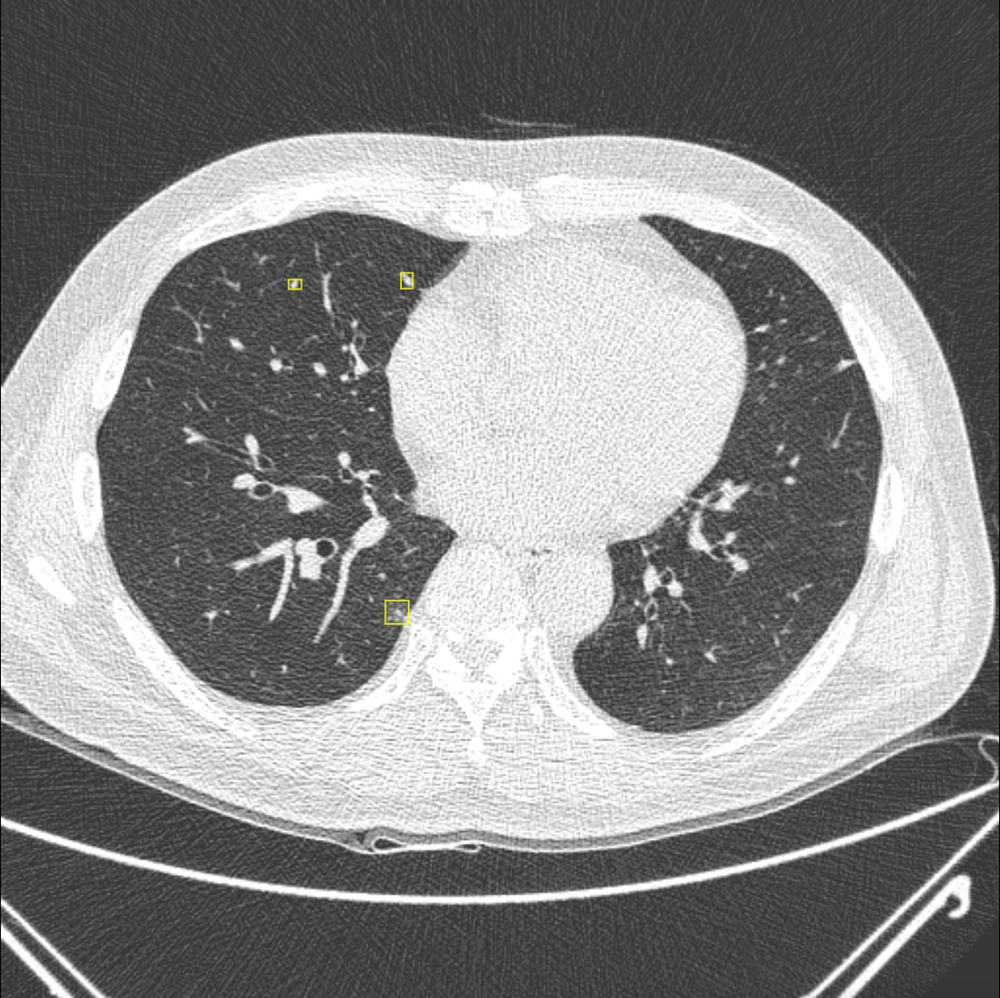Before proceeding, let’s clearly specify the problem. We use the following terminology:

• Case A(n) (set of) image(s) from a patient. For example, an anterior-posterior and lateral chest X-ray, a CC/MLO mammogram or a color fundus scan.

Each case consists of a set of findings (which can be the empty set), that are defined by the human annotators and a set of detections (that can also be the empty set), which are generated by the detection algorithm. Both are described below.

• Finding A polygon annotation for a sign of a disease. For example, a contour around a set of microcalcifications in a mammogram or a bounding box (a specific type of polygon) around a chest nodule. In the remainder of this post, the term finding is used interchangeably with the term ‘lesion’.

• Detection The output of our detection system (for example a deep convolutional neural network based architecture like YOLO or faster R-CNN), zero or more for every case.

The detection typically has two degrees of freedom (x, y) or four degrees of freedom (x, y, width, height). The latter is more common in modern detection architectures. These definitions can easily be extended to 3D where we have (x, y, z) or (x, y, z, width, height, depth).

In addition to the location, each detection comes with a scalar score (corresponding to a binary output), that is some indication of the degree of suspicion that the model assigns to the detection (for example a score of 0.8 that a location contains a malignant lesion). This value is often between 0 and 1, but nothing that is discussed below will require it to be in that range.

We have our cases evaluated by our model and want to know how good the model is at detecting the findings for this set of cases. A perfect system would detect all and only the annotations at the exact location and rate them the highest possible score.

The ROC curve

The ROC is a curve typically used to evaluate classification models. These models only output a single score for a case (with one degree of freedom), instead of a set of detections. The ROC curve plots

• X-axis Case level (1 — specificity) or false positive rate

• Y-axis Case level sensitivity or true positive rate

There are (at least) two ways in which we can use the ROC curve to evaluate detections in a case, both of these have some limitations:

1. Ignore the case structure and only look at the lesions

In this setting, all the boxes that hit (we will go into details on what exactly a hit is later on) the ground truth and have a score above the threshold are true positives, all boxes that do not hit the ground truth and have a score above the threshold are false positives, etc.

A key problem with this is that negatives depend strongly on the model, so every time the model changes, the distribution of samples changes. The area under the ROC is invariant to class proportion, but not to distribution (though there are transformations that would change the shape of the distribution of the outputs, but not the AUC). This means different models will be hard to compare. For example, decreasing a non-maximum suppression threshold will yield many boxes, which could be easy to classify, this means the distributions are easier to separate and the area under the curve (AUC) will go up, even though the visual results will be poor.

Secondly and slightly related, the value is difficult to interpret. Since the model can determine its own ‘true negatives’, an AUC value of 0.99 can mean a very good model or a model with many easy ‘true negatives’. To better understand this, figure 2 provides an illustration of scores output by a model. As long as the positives and negatives are sampled from the same distribution, the ROC curve will be the same (in the limit). However, when the distribution of one of the two changes, this can lead to deceptively simple separation.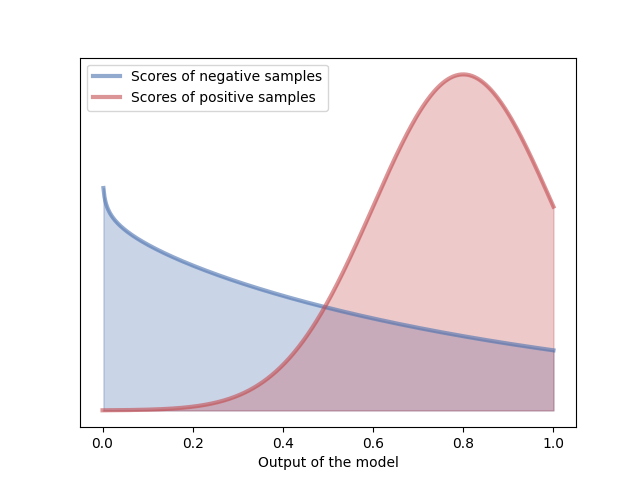2. Ignore the lesion structure and only look at cases

In this setting, some aggregation operation over all the detected boxes in a case is used to get a score for every single case. This is typically the box with the maximum predicted score (i.e., the max operator). However, this does not capture the performance of the model accurately; similar models would be mapped to the same score.

These problems are depicted in figure 4 and explained below in detail:

1. The case level ROC does not measure if the lesion is actually hit or not.
If the model detects a lesion in the top left of the case, but the actual finding is in the bottom right corner, the case is still counted as a true positive if the score is above the threshold.

2. The case level ROC is invariant to the number of annotated lesions in the case. The curve can not discriminate between a model detecting all 10 lesions in a case or just detecting 1 of them, as long as the maximum score of all lesions is the same.

3. The case level ROC is invariant to the number of detections in the case.
Similarly, the curve can not discriminate between a model that detects 10 false positives in a case or 1 false positive, as long as the maximum score is the same. This can be a serious problem for object detection models which ,unlike humans, typically do not have prior knowledge about the number of lesions to be expected in a case. A poorly calibrated non-maximum suppression threshold in a faster R-CNN-like model for example, can yield several detections for the same finding or hundreds of boxes for a case.

Note that similar problems hold for different aggregation operators than the max operator. To prevent some of these problems, several alternatives have been investigated, which are essentially different combinations of the ROC on the lesion level and the ROC on a case level, which will be discussed below.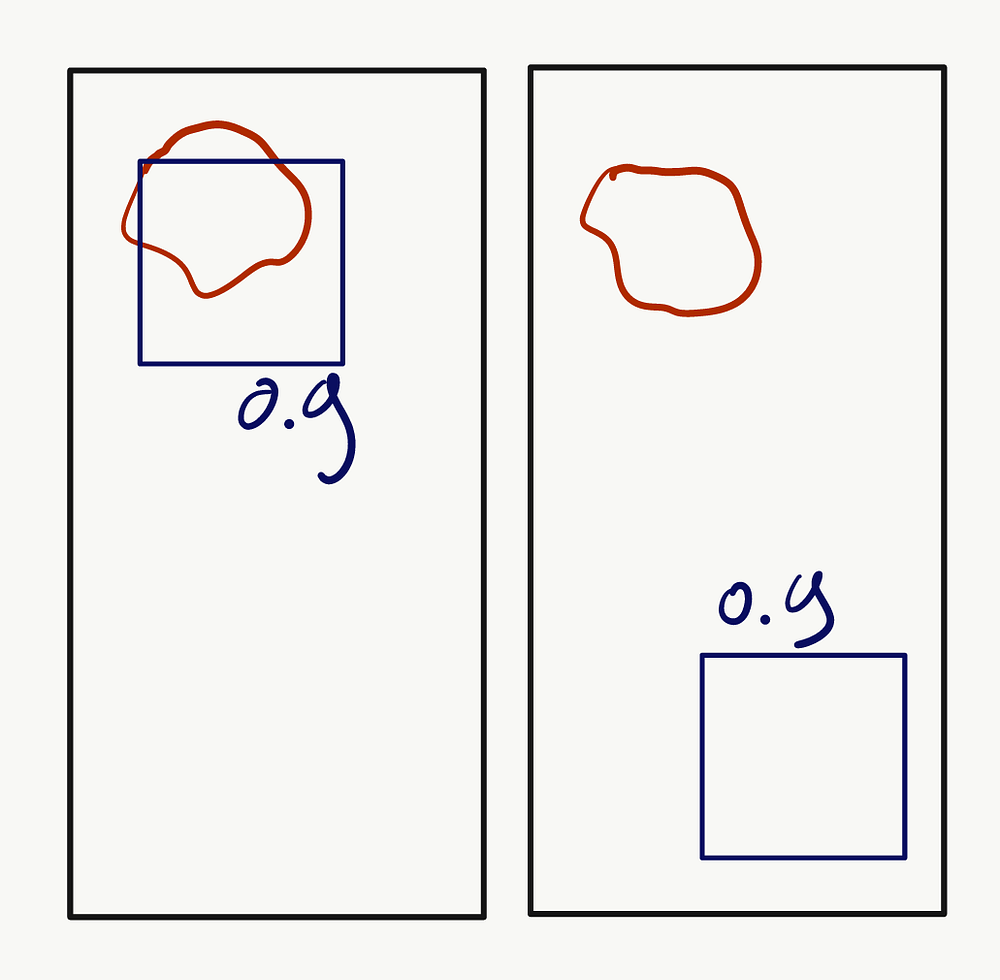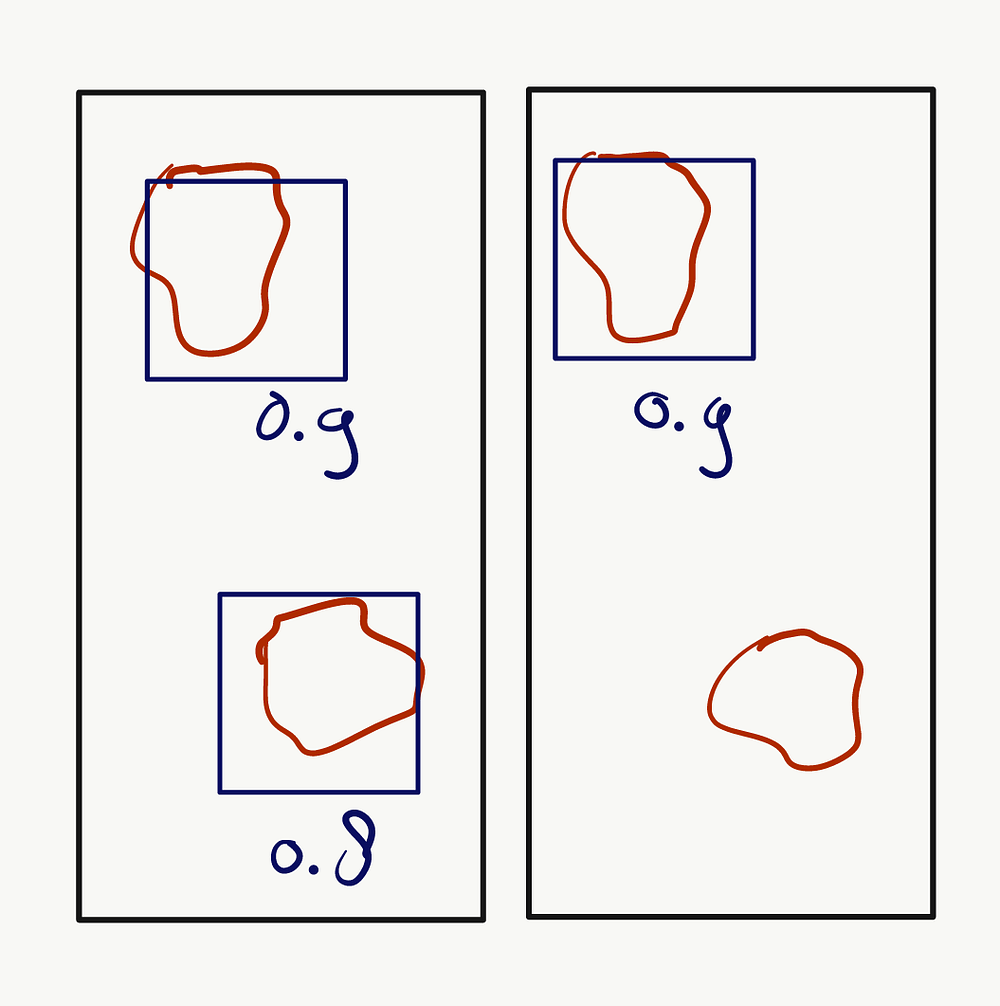Localization ROC

One way to change the case level ROC curve to tackle problem 1 of using the ROC on a case level, is to require the detection to also hit the annotation (again, what a hit is is described later). This results in the LROC or localization ROC curve, which plots

• X-axis Case level (1 — specificity) or false positive rate

• Y-axis Case level probability of correct localization or the fraction of cases with a true positive that also correctly hits the annotated lesion.

The LROC is essentially the same as the ROC on a case level, except that we require it to hit the finding. This means that the sensitivity does not necessarily go up to 1 as not all lesions may be hit.

Although the LROC solves the first problem that the case-level ROC has, it does not solve problems 2 and 3. The default version of the LROC (without any further assumptions on the type of data) does not consider multiple annotated lesions in an image and does not consider multiple false positives. Though it is possible to add a constraint that requires it to hit ALL findings, not ANY finding, this again will have a similar problem. The model will not be able to tell the difference between hitting just one of the findings or a model not hitting any.

FROC

One solution to the 2nd and 3rd problem of using the ROC on a case level is the free response operator characteristic curve or FROC curve. This curve plots:

• X-axis The average number of false positives per case.

• Y-axis Lesion localization fraction (LLF) or lesion level sensitivity

Instead of looking at the data on a case level, the FROC looks at the data on a lesion level, meaning it does not suffer from problems 2 and 3. It can discriminate between multiple findings per case and multiple false positives. The FROC also has the nice property that it is simple to read off the false positives per case from the X-axis, which is often important in the clinic.

One problem with this curve, however, is that the area under the curve, a common summary statistic, is not bounded between 0 and 1. Since we can have many findings in a specific case, the AUC can go up to infinity (in theory). This property makes doing statistical analysis complicated because common assumptions of tests do not hold.

It also makes comparison between two models that are not evaluated on the same dataset difficult, because a few cases with many false positives can strongly influence the area under the FROC.

Logarithmic scaling of FROC

When computer aided detection systems are used in the real world, we are often interested in a very low number of false positives. A system that catches everything that can possibly be a sign of disease, but has 10 false positives is typically not useful as a detection aid. One way to emphasize prioritizing high specificity models and simultaneously ‘zoom in’ on relevant false positive rates is to scale the X-axis logarithmically. This has been applied to nodule detection in CT  and lesion detection in mammograms . Using this type of scaling however, models with differently shaped ROCs, but the same AUC can be mapped to different area under the FROC.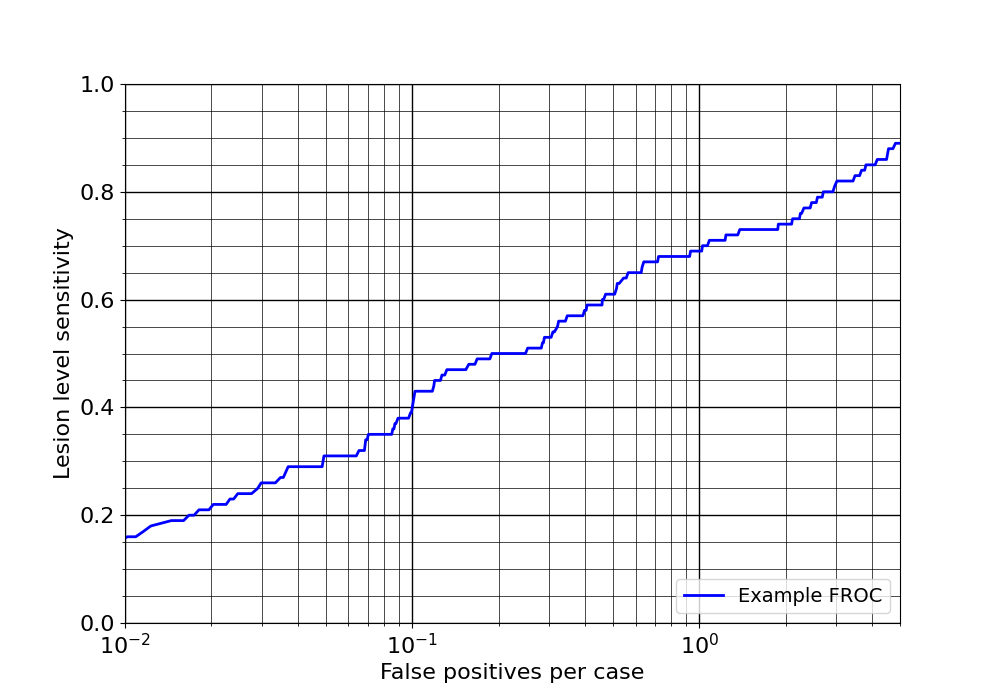Partial AUC of the FROC

Another way to narrow down on a subset of operating points is by integrating the curve around a desired false positive rate or false positives per case, for example 0.001 to 0.1, meaning we are only interested in a system that gives a false positive 1 in a 1000 cases to 1 in 10 cases.

Using the partial area under the FROC also bounds the performance to [0, 1], making it easy to compare models. This can be used in combination with the logarithmic scaling . An example of an FROC curve with logarithmic scaling on the X-axis is shown in figure 5.

AFROC/JAFROC

Another curve that solves the problem of the unbounded AUC of the FROC is the alternative free response operator characteristic or AFROC curve. The AFROC curve is usually referred to as JAFROC (short for Jackknifed AFROC), however, this is a misnomer. The jackknife is a statistical sampling technique that is used to generate confidence intervals and compare the curves of different models, often used in combination with the AFROC curve. Other techniques such as the bootstrap could also be used . Or as is written in the official documentation: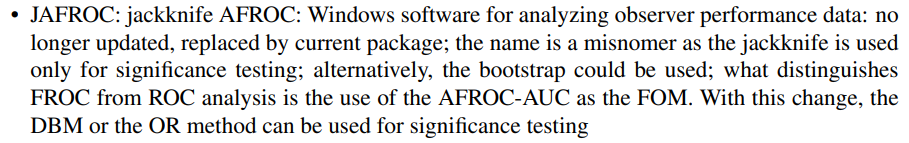The AFROC curve plots

• X-axis The case level (1 — specificity) or false positive rate

• Y-axis The lesion localization fraction or lesion level sensitivity

This essentially takes the Y-axis of the FROC and X-axis of the ROC/LROC. Although the area under the curve is now bounded between 0 and 1, it again suffers from problem 3: a model that detects 10 false positives in a case will have the same area under the AFROC as a model that detects only 1 false positive in the same case, as long as the highest score of all the detections is the same.

Weighted FROC and AFROC

One more limitation of the FROC and AFROC curves is that cases with more lesions will have a high weight in the final aggregate statistic. This is not always desirable, sometimes specific annotators may have annotated too many lesions in a case, sometimes a disease subtype manifests itself in the form of multiple lesions, etc. A simple way to prevent this is to weigh the lesions according to the number of findings in the scan. The weighting scheme can also be used to emphasize more clinically relevant lesions.

### Hit criteria

The curves described above are applicable when we already have a set of lesions and a set of detections that hit the findings and that missed the findings. However, we still need to define what a hit and miss is exactly. This is where the hit criterion is used, which is sometimes referred to as ‘mark labelling’ .

The hit criterion takes as input a case with some annotated findings and a set of detections output by the model. It outputs a binary value for each finding that indicates if the finding is hit or not. Similarly, for each detection it outputs a binary value indicating if it hits a finding or not. This gives us a set of true positives, false positives, true negatives and false negatives from which we can generate the curves described above.

Lesion center cover criterion

One hit criteria that is sometimes used to determine if a lesion is hit, is if the detection covers the center of the annotated lesion. A lesion is considered hit if the center of the lesion is inside the detection. An obvious downside of this though, is that a model can output massive boxes to maximize the chance of a detection, which is illustrated in figure 6.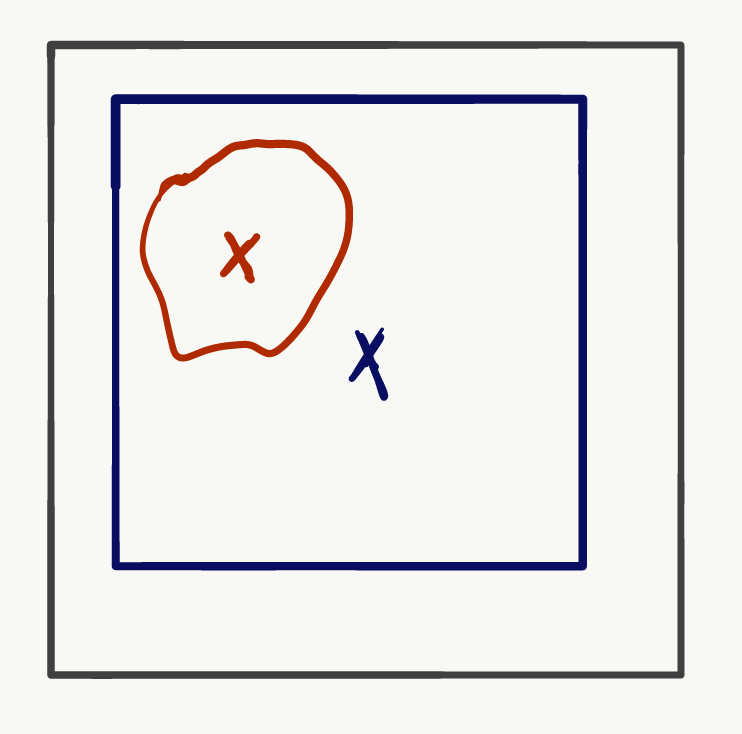Distance based hit criterion

Another commonly used hit criterion is the distance based hit criterion. The lesion is considered hit if the distance between the center of the detection and center of the lesion is smaller than a threshold.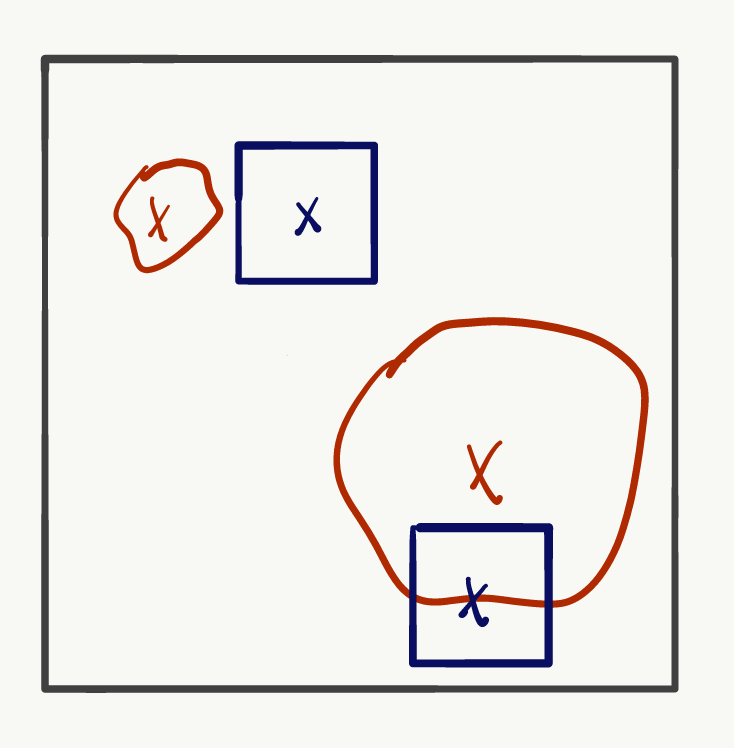A problem with this criterion is that large and small lesions are treated equally, which can result in poor visual performance. This is illustrated in figure 7, the distance to the center of both lesions (red circles) is the same, but visually the results look poor as the top left lesion does not have any overlap with the detection. One way to solve this is to normalize the threshold by the size of the lesion as was done in the LUNA16 challenge .

A second problem with this hit criterion is that it does not review all the degrees of freedom of a model, which is illustrated in figure 8. As long as the center of the box is inside the annotated area, the lesion is considered hit, regardless of the shape and size of the detection.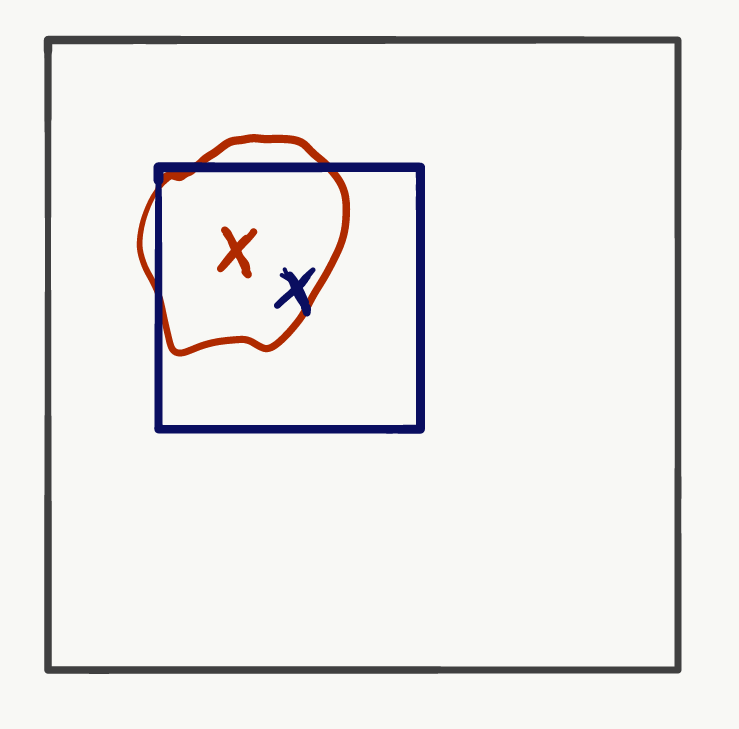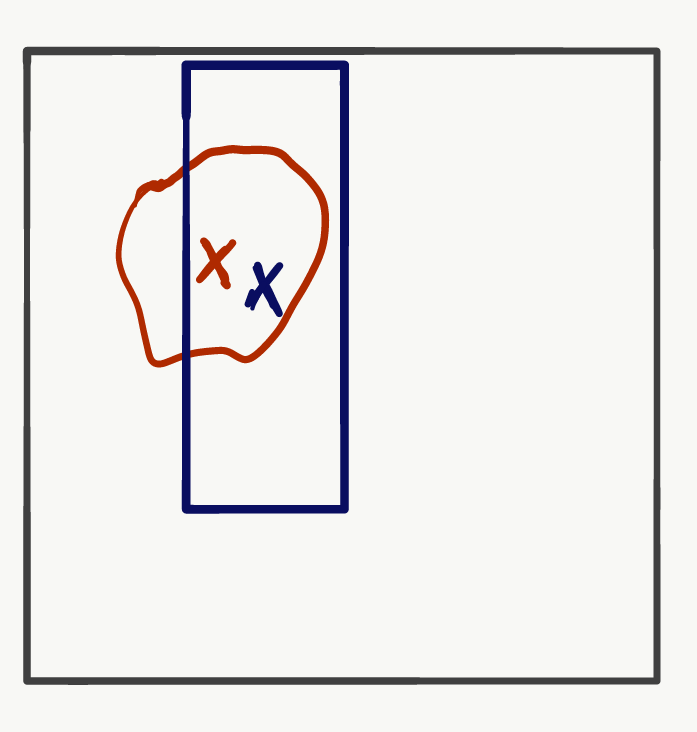Center-hit criterion

One more commonly used criterion looks at the center of the detection. The lesion is considered hit if the center of the detection is inside the annotated contour or bounding box. Similar to the distance based criterion, this does not consider all degrees of freedom of the detection: the two boxes in figure 8 are both correct. A second problem is that larger lesions are more likely to be hit, which is illustrated in figure 9.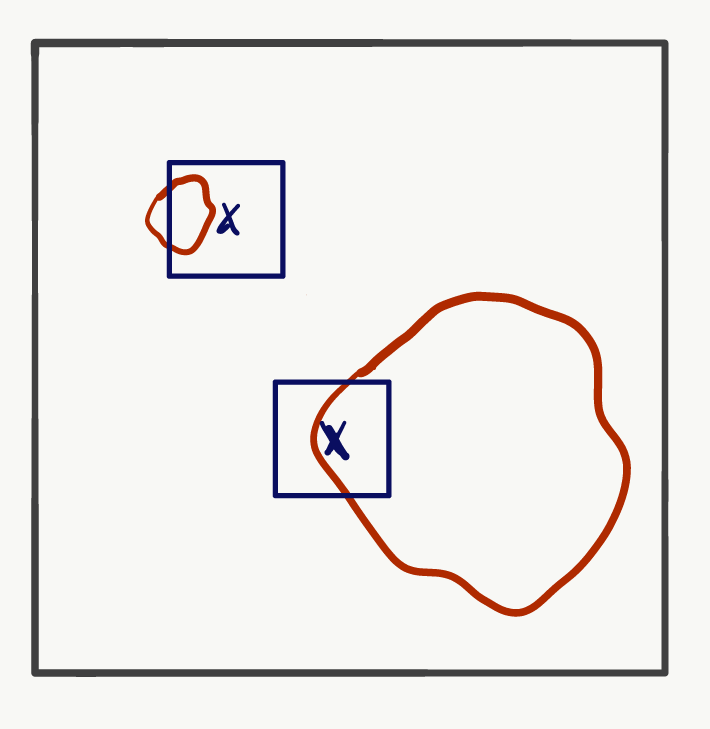Intersection over union

The intersection over union (IoU) is scale invariant and therefore does not bias large or small lesions. The model can also not cheat by just generating very large boxes, because the IoU will be small. Thirdly, all degrees of freedom of the detection are evaluated as it will penalize badly overlapping regions.

Summary

To summarize, a good evaluation for detection models (with four degrees of freedom) may be the partial FROC, that is bounded to [0, 1], integrated around a clinically relevant operating point. As a hit criterion, the IoU might be suitable, as it evaluates all degrees of freedom that are output by modern meta-architectures (such as Faster R-CNN and YOLO) and is not biased towards any particular lesion size, though it does come with other problems .

In the end the optimal evaluation metrics are highly problem specific. For some applications it could be sufficient if we detect at least one of the abnormalities in a case, for example if one abnormality would already warrant follow-up examination (for model diagnosis though, we would still want more granularity), for some we need to detect all. For some applications only one abnormality can exist in a case (typically abnormalities related to body parts for which we only have one, such as mediastinum, heart, etc). Not all of the pros and cons of the curves and hit criteria mentioned above may be relevant for all problems.

Coming up with a good way to measure how well your solution solves a problem is challenging and requires integrating domain knowledge with technical knowledge. Picking the best metric is similar to picking a loss function for your statistical model: it requires analyzing the problem, talking to experts and lots of careful considerations.

Acknowledgements

Many thanks to Sergio, Jongchan and Seonwook for their comments on the post and fruitful discussions.

Part of this post will be integrated in the paper:

Reinke, A., Eisenmann, M., Tizabi, M.D., Sudre, C.H., Rädsch, T., Antonelli, M., Arbel, T., Bakas, S., Cardoso, M.J., Cheplygina, V. and Farahani, K., 2021. Common limitations of image processing metrics: A picture story. arXiv preprint arXiv:2104.05642.

which collects common pitfalls for the evaluation of segmentation and detection systems.

References

1. Bunch, P.C., Hamilton, J.F., Sanderson, G.K. and Simmons, A.H., 1978. A free-response approach to the measurement and characterization of radiographic-observer performance. J. Appl. Photogr. Eng, 4(4), pp.166–171.

2. Chakraborty, D.P. and Zhai, X., 2016. On the meaning of the weighted alternative free‐response operating characteristic figure of merit. Medical physics, 43(5), pp.2548–2557.

3. Chakraborty, D.P. and Berbaum, K.S., 2004. Observer studies involving detection and localization: modeling, analysis, and validation. Medical physics, 31(8), pp.2313–2330.

4. Swensson, R.G., 1996. Unified measurement of observer performance in detecting and localizing target objects on images. Medical physics, 23(10), pp.1709–1725.

5. Chakraborty, D.P., 2013. A brief history of free-response receiver operating characteristic paradigm data analysis. Academic radiology, 20(7), pp.915–919.

6. Petrick, N., Sahiner, B., Armato III, S.G., Bert, A., Correale, L., Delsanto, S., Freedman, M.T., Fryd, D., Gur, D., Hadjiiski, L. and Huo, Z., 2013. Evaluation of computer‐aided detection and diagnosis systems a. Medical physics, 40(8), p.087001.

7. Sun, P., Zhang, R., Jiang, Y., Kong, T., Xu, C., Zhan, W., Tomizuka, M., Li, L., Yuan, Z., Wang, C. and Luo, P., 2021. Sparse r-cnn: End-to-end object detection with learnable proposals. In Proceedings of the IEEE/CVF Conference on Computer Vision and Pattern Recognition (pp. 14454–14463).

8. Fawcett, T., 2006. An introduction to ROC analysis. Pattern recognition letters, 27(8), pp.861–874.

9. Redmon, J., Divvala, S., Girshick, R. and Farhadi, A., 2016. You only look once: Unified, real-time object detection. In Proceedings of the IEEE conference on computer vision and pattern recognition (pp. 779–788).

10. Ren, S., He, K., Girshick, R. and Sun, J., 2015. Faster r-cnn: Towards real-time object detection with region proposal networks. Advances in neural information processing systems, 28, pp.91–99.

11. R JAFROC manual https://cran.r-project.org/web/packages/RJafroc/RJafroc.pdf

12. Kallergi, M., Carney, G.M. and Gaviria, J., 1999. Evaluating the performance of detection algorithms in digital mammography. Medical Physics, 26(2), pp.267–275.

13. Kooi, T., Litjens, G., Van Ginneken, B., Gubern-Mérida, A., Sánchez, C.I., Mann, R., den Heeten, A. and Karssemeijer, N., 2017. Large scale deep learning for computer aided detection of mammographic lesions. Medical image analysis, 35, pp.303–312.

14. Setio, A.A.A., Traverso, A., De Bel, T., Berens, M.S., van den Bogaard, C., Cerello, P., Chen, H., Dou, Q., Fantacci, M.E., Geurts, B. and van der Gugten, R., 2017. Validation, comparison, and combination of algorithms for automatic detection of pulmonary nodules in computed tomography images: the LUNA16 challenge. Medical image analysis, 42, pp.1–13

15. Reinke, A., Eisenmann, M., Tizabi, M.D., Sudre, C.H., Rädsch, T., Antonelli, M., Arbel, T., Bakas, S., Cardoso, M.J., Cheplygina, V. and Farahani, K., 2021. Common limitations of image processing metrics: A picture story. arXiv preprint arXiv:2104.05642.

AIArtificial IntelligenceDeep LearningLunitMachine LearningRadiologyResearch